Multiple lines of symmetry

Chapter 12 Class 7 Symmetry
Concept wise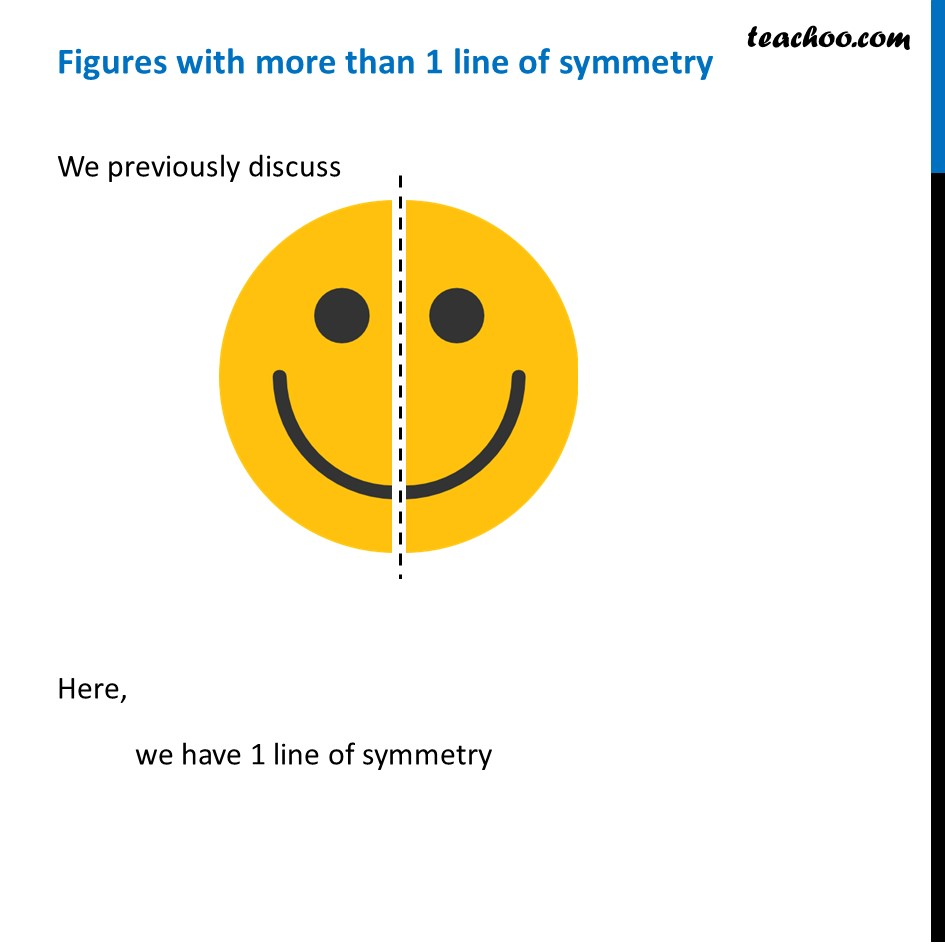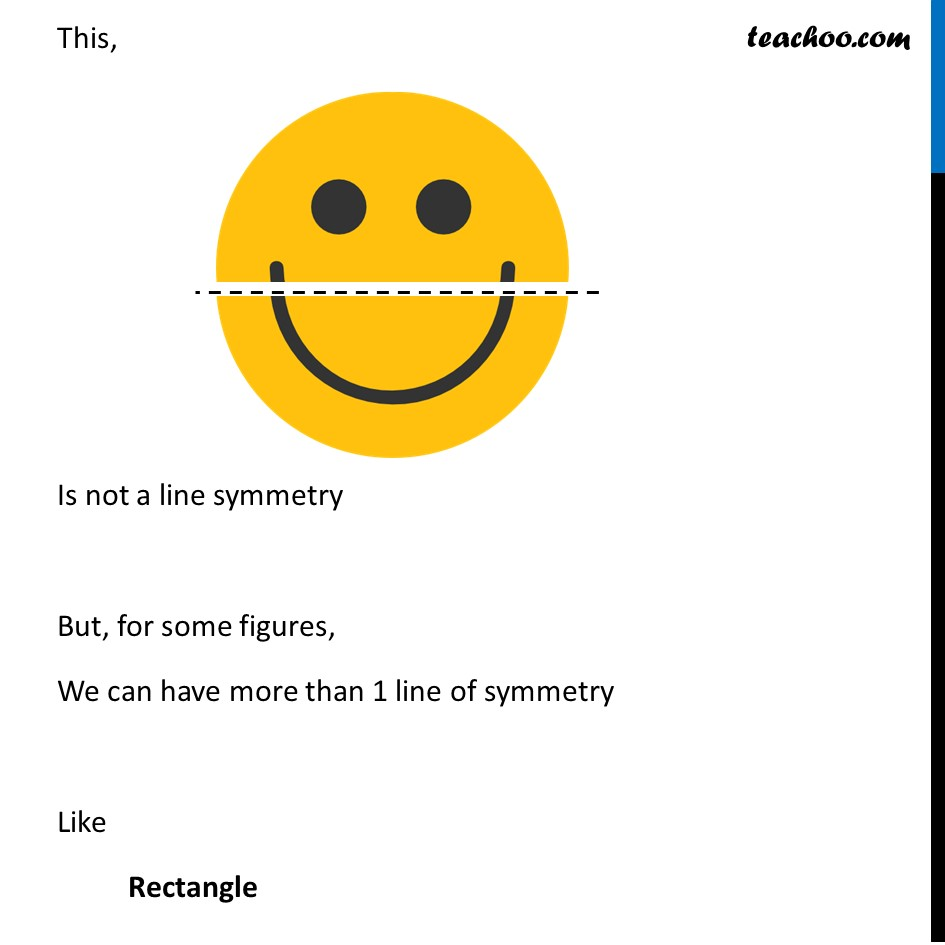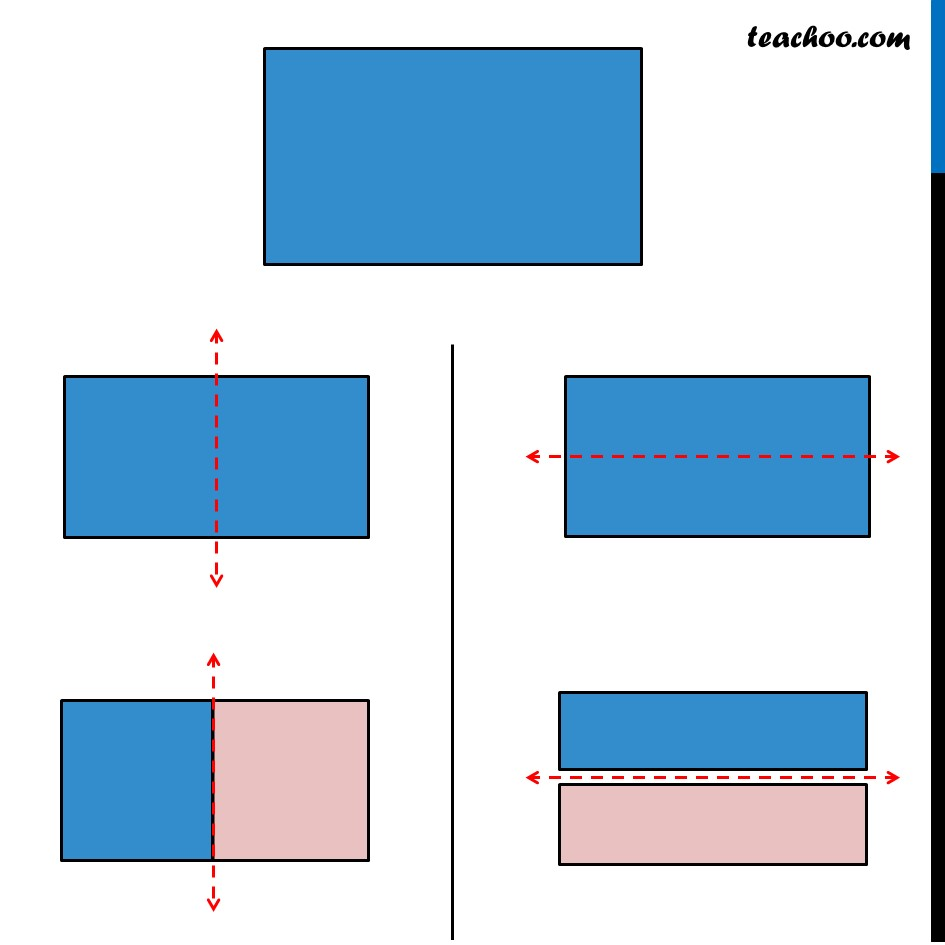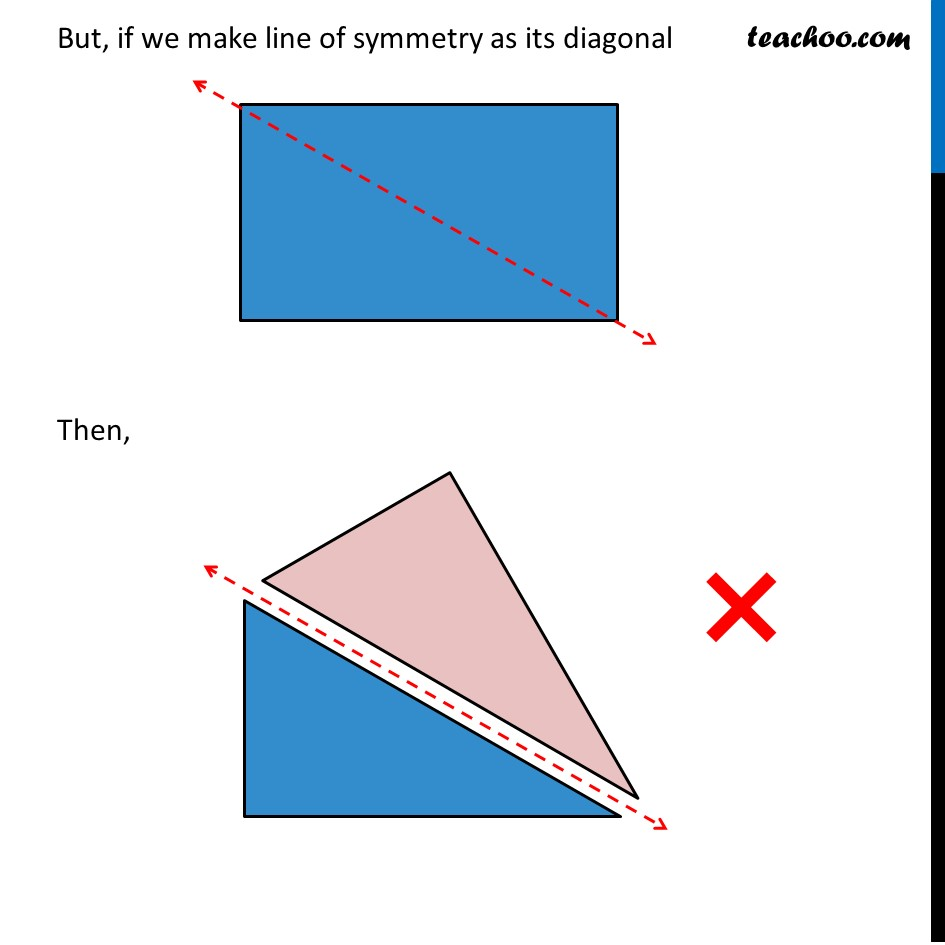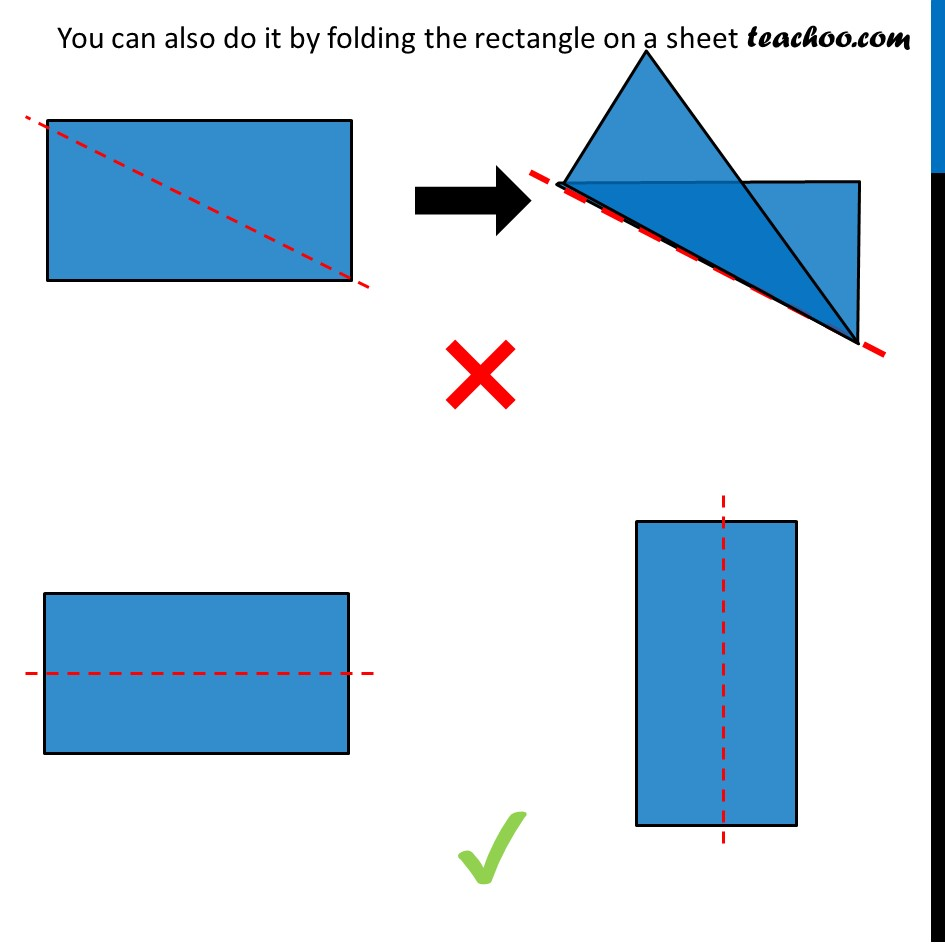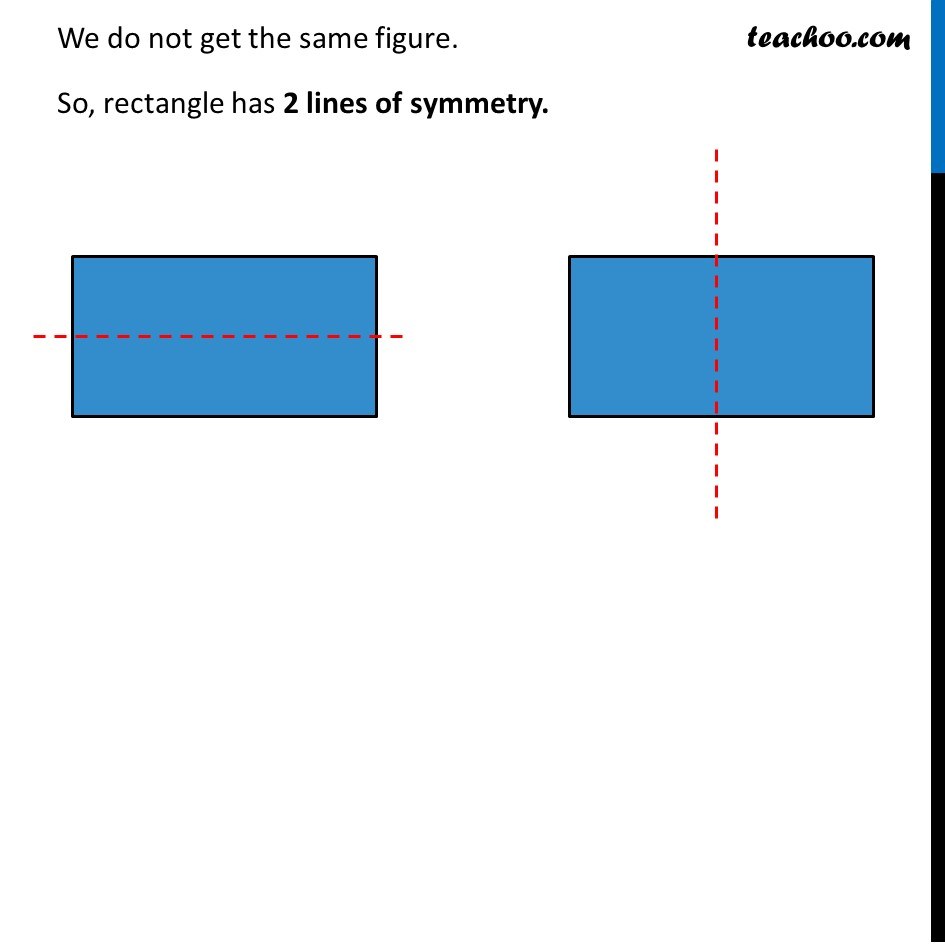Learn in your speed, with individual attention - Teachoo Maths 1-on-1 Class

### Transcript

Figures with more than 1 line of symmetryWe previously discuss Here, we have 1 line of symmetry This, Is not a line symmetry But, for some figures, We can have more than 1 line of symmetry Like Rectangle But, if we make line of symmetry as its diagonal Then, You can also do it by folding the rectangle on a sheet We do not get the same figure. So, rectangle has 2 lines of symmetry. Figures with more than 1 line of symmetryWe previously discuss Here, we have 1 line of symmetry This, Is not a line symmetry But, for some figures, We can have more than 1 line of symmetry Like Rectangle But, if we make line of symmetry as its diagonal Then, You can also do it by folding the rectangle on a sheet We do not get the same figure. So, rectangle has 2 lines of symmetry.